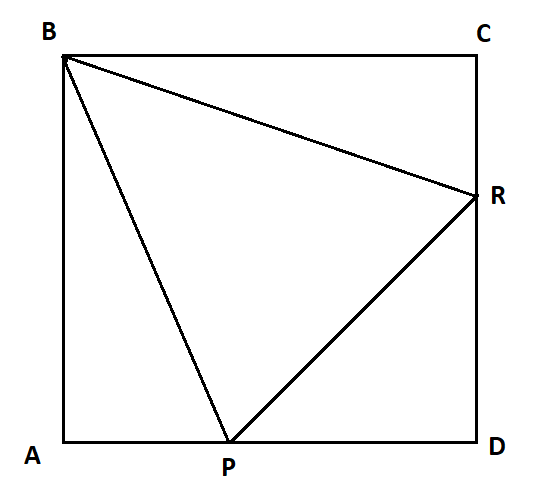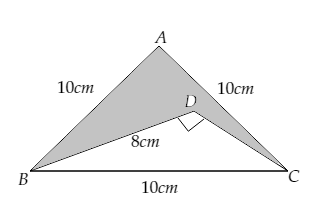Filters
Latest Questions
CBSE
Mathematics
Properties of equilateral triangle
Given the equilateral triangle inscribed in a square of side s find the ratio of $\Delta BCR$ to $\Delta PRD$ ?CBSE
Mathematics
Properties of equilateral triangle
Can an equilateral triangle be a right triangle?
CBSE
Mathematics
Properties of equilateral triangle
The length of each side of an equilateral triangle is increased by $5$ inches, so, the perimeter is now $60$inches. How do you write and solve an equation to find the original length of each side of the equilateral triangle?
CBSE
Mathematics
Properties of equilateral triangle
In a quadrilateral $ABCD$, which is not a trapezium. It is known that $\angle DAB = \angle ABC = 60^\circ$. Moreover, $\angle CAB = \angle CBD$. Then
A. $AB = BC + CD$
B. $AB = AD + CD$
C. $AB = BC + AD$
D. $AB = AC + AD$?
CBSE
Mathematics
Properties of equilateral triangle
Why are all equilateral triangles acute?

CBSE
Mathematics
Properties of equilateral triangle
In the given figure, $\vartriangle ABC$ is an equilateral triangle the length of whose side is equal to $10$ cm, and $\vartriangle DBC$ is right-angled at D and BD $= 8$ cm. Find the area of the shaded region. [Take $\sqrt 3 = 1.732$ ]CBSE
Mathematics
Properties of equilateral triangle
Find the area of the equilateral triangle having length of each side 50 cm.
CBSE
Mathematics
Properties of equilateral triangle
Prove that the area of an equilateral triangle described on one side of the square is equal to half the area of the equilateral triangle described on one of its diagonal.
CBSE
Mathematics
Properties of equilateral triangle
Find the measure of each angle of an equilateral triangle.
CBSE
Mathematics
Properties of equilateral triangle
ABC and BDE are two equilateral triangles such that “D” is the midpoint of BC. Ratio of the area of the triangles ABC and BDE is
A.$2:1$
B.$1:4$
C.$1:2$
D.$4:1$

CBSE
Mathematics
Properties of equilateral triangle
What‌ ‌is‌ ‌the‌ ‌area‌ ‌of‌ ‌an‌ ‌equilateral‌ ‌triangle‌ ‌with‌ ‌height‌ ‌of‌ ‌9‌ ‌inches?‌ ‌

CBSE
Mathematics
Properties of equilateral triangle
What is the area of an equilateral triangle with a side length of $5?$

Prev
1
2
3
4
5